We recommend switching to the latest versions of Edge, Firefox, Chrome or Safari. Using Internet Explorer will result in a loss of website functionality.

•Since the forum does not permit LNA files to be posted here, I have written up a Help Center post where you can find the code.  Even so, here are the details of that example.

The example uses the Transform node to output multiple records for a single input record. The records could be published to a file using the Output Delimited node, as usual.

The data flow is as follows: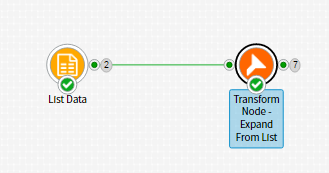The data in the Static Data node is:

account:int,state list:string
11111111,"FL, MA, NE"
22222222,"CA, AZ, NV, NM"

In this case the Transform node has been configured with some custom properties (defined in the 'Define' tab of the node's configuration panel) :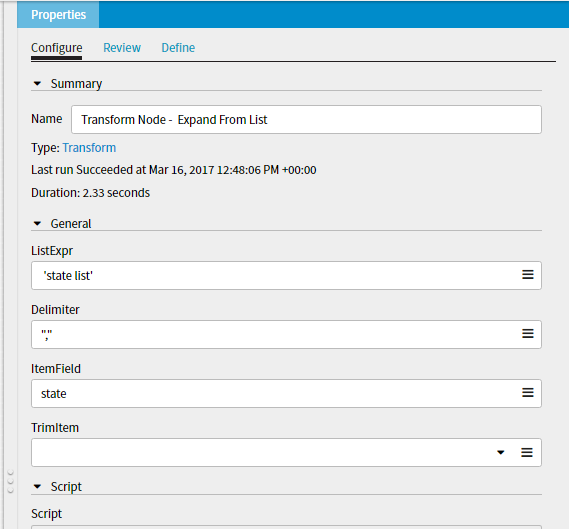Define tab view: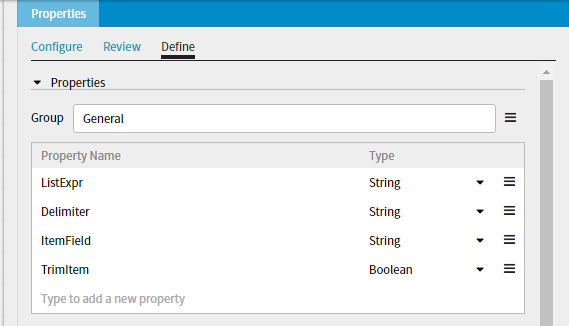The Script property of the Transform node is configured with the logic to generate the required output: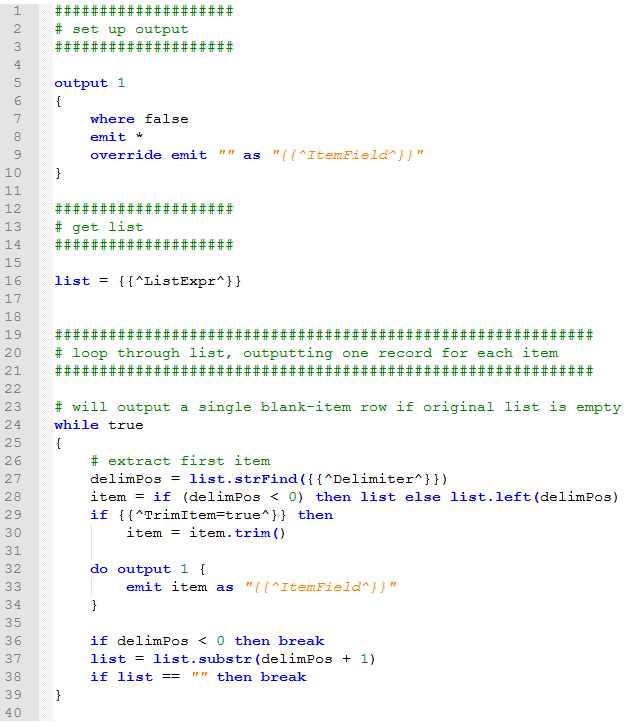The actual script is included at the end of this post.

The output data is: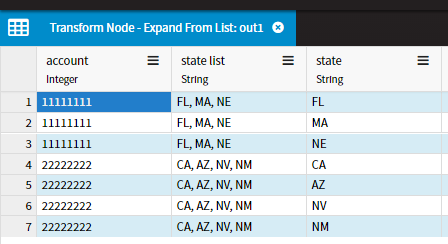Regards,

####################
# set up output
####################

output 1
{
where false
emit *
override emit "" as "{{^ItemField^}}"
}

####################
# get list
####################

list = {{^ListExpr^}}

############################################################
# loop through list, outputting one record for each item
############################################################

# will output a single blank-item row if original list is empty
while true
{
# extract first item
delimPos = list.strFind({{^Delimiter^}})
item = if (delimPos < 0) then list else list.left(delimPos)
if {{^TrimItem=true^}} then
item = item.trim()

do output 1 {
emit item as "{{^ItemField^}}"
}

if delimPos < 0 then break
list = list.substr(delimPos + 1)
if list == "" then break
}

•Thank you!

How is this compares to having one source node sending outputs to multiple transform nodes (in terms of performance and other considerations)?

Also, if I use multiple transform nodes, is there a way to merge the output back to a single file?

•Dear Shu,

For your first question, I presume you are talking about a situation similar to the one described here.  In that case, I would say the following:

• The first approach--the one with the three nodes--is easier to understand, but the code will mostly likely run more slowly as the input data has to be read in three times.
• The second approach is a bit more complicated but will most probably run faster since the node only has to run over the input data once.

Other than that, there are very few considerations.

Hope that helps,

Rocco

•Look forward to your insights on the second question. Cheers.

•Hi Shu,

I just realized that we did not answer your second question.  If you have multiple data sets that you want to add together into a single data set you would use the Cat node.  This node will concatenate the data from each input into a single output.  If the fields are the same for all inputs then no configuration is needed.  If the fields from the inputs are different then you will need to set the ConcatenationMode parameter on the Cat node to either Union or Intersection.

Regards,

Larry